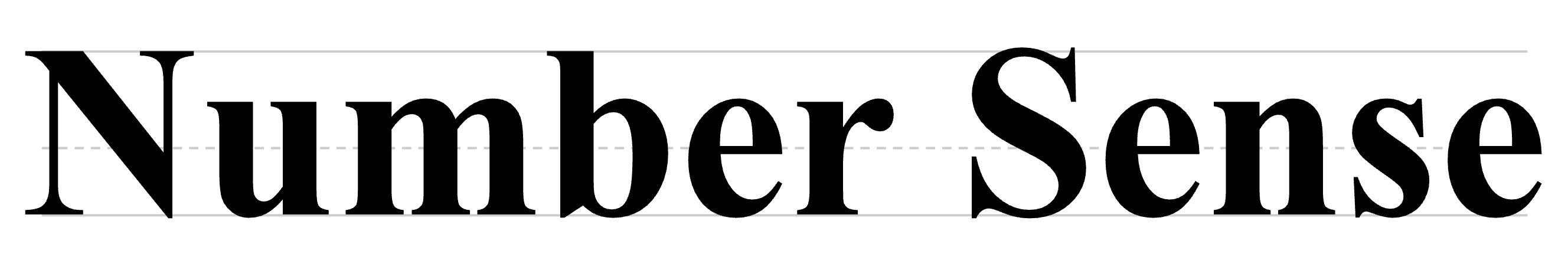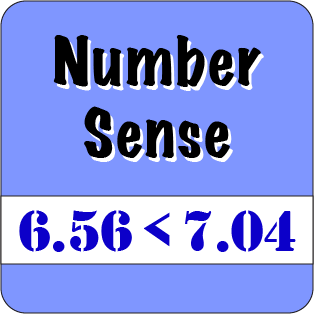Back to Mr. Anker Tests, Grade 4Place Value
 Place Value 5 Identify the place value of digits in numbers up to the ten thousands. Place Value 6 Identify the place value of digits in numbers up to the hundred thousands. Place Value 7 Identify the place value of digits in numbers up to the ten millions. Place Value 8 Identify the place value of digits in numbers up to the hundred millions.

Rounding to the Nearest Ten, Hundred, Thousand, and Beyond
 Rounding tothe Nearest Ten 1 Multiple Choice with hints. Rounding tothe Nearest Ten 2 Type in the Answer. Rounding to Nearest Hundred 1 Multiple Choice with hints. Rounding to Nearest Hundred 2 Type in the Answer.

Standard & Expanded Form
 Standard & Expanded Form 5 Numbers under 1,000,000 & fill in missing numbers. Standard & Expanded Form 6 Numbers under 1,000,000 & fill in missing numbers. Standard & Expanded Form 7 Numbers into the hundred millions & fill in missing numbers. Standard & Expanded Form 8 Numbers into the billions & fill in missing numbers.

Writing Numbers and Larger Numbers
 Writing Numbers 1 Numbers under 500 Writing Numbers 2 Numbers under 100,000 Writing Large Numbers 3 Numbers up to one million Writing Larger Numbers 4 Numbers into the hundred millions

Greater Than, Less Than, Equal To
 Greater, Less Than 5 Grades 1-2Numbers under 100 Greater, Less Than 6 Grades 2-3Numbers under 1,000 Greater, Less Than 7 Grade 3-4Numbers under 10,000 Greater, Less Than 8 Grade 4Numbers up to 1 billion

Prime Factorization
 Prime Factorization 1Algebraic Thinking 1.4 Determine all the prime factors through 50 and write the numbers as a product of their prime factors, including exponents. Prime Factorization 2Algebraic Thinking 1.4 Determine all the prime factors through 50 and write the numbers as a product of their prime factors, including exponents. Prime Factorization 3Algebraic Thinking 1.4 Determine all the prime factors through 50 and write the numbers as a product of their prime factors, including exponents. Prime Factorization 4Algebraic Thinking 1.4 Determine all the prime factors through 50 and write the numbers as a product of their prime factors, including exponents.
 Prime Factorization 5Algebraic Thinking 1.4 Determine all the prime factors through 50 and write the numbers as a product of their prime factors, including exponents.

Attributes of Numbers
 Finding the Range 1 Identify features of data sets, including range, mode, median & outlier. Finding the Range 2 Identify features of data sets, including range, mode, median & outlier. Finding the Range 3 Identify range with decimal values. Finding the Range 4 Identify range with negative values.
 Finding the Median 1 Identify features of data sets, including range, mode, median & outlier. Finding the Median 2 Identify features of data sets, including range, mode, median & outlier. Finding the Median 3 Identify features of data sets, including range, mode, median & outlier. Finding the Mode 1 Identify features of data sets, including range, mode, median & outlier.
 Finding the Outlier 1 Identify features of data sets, including range, mode, median & outlier. Odd/Even Numbers Determine if a number is odd or even.

Exponents
 Exponents 1 Know and apply the properties of integer exponents to generate equivalent numerical expressions. Exponents 2 Know and apply the properties of integer exponents to generate equivalent numerical expressions. Exponents 3 Know and apply the properties of integer exponents to generate equivalent numerical expressions. Exponents 4 Know and apply the properties of integer exponents to generate equivalent numerical expressions.
 Exponents 5 Know and apply the properties of integer exponents to generate equivalent numerical expressions.

Square Roots
 Square Roots 1 Evaluate square roots of small perfect squares and cube roots of small perfect cubes. Square Roots 2 Evaluate square roots of small perfect squares and cube roots of small perfect cubes. Square Roots 3 Use square root and cube root symbols to represent solutions to equations of the form x2 = p and x3 = p, where p is a positive rational number Square Roots 4 Use square root and cube root symbols to represent solutions to equations of the form x2 = p and x3 = p, where p is a positive rational number
 Square Roots 5 Use square root and cube root symbols to represent solutions to equations of the form x2 = p and x3 = p, where p is a positive rational number Square Roots 6 Use square root and cube root symbols to represent solutions to equations of the form x2 = p and x3 = p, where p is a positive rational number Square Roots 7 Use square root and cube root symbols to represent solutions to equations of the form x2 = p and x3 = p, where p is a positive rational number Square Roots 8 Use square root and cube root symbols to represent solutions to equations of the form x2 = p and x3 = p, where p is a positive rational number
 Square Roots 9 Use square root and cube root symbols to represent solutions to equations of the form x2 = p and x3 = p, where p is a positive rational number

Absolute Value
 Absolute Value 1 Understand the absolute value of a rational number as its distance from 0 on the number line... Absolute Value 2 Understand the absolute value of a rational number as its distance from 0 on the number line... Absolute Value 3 Understand the absolute value of a rational number as its distance from 0 on the number line... Absolute Value 4 Understand the absolute value of a rational number as its distance from 0 on the number line...

Equalities & Inequalities
 Equalities & Inequalities 1 Insert <, >, =, +, -, x, ÷, and values into number sentences. Equalities & Inequalities 2 Insert <, >, =, +, -, x, ÷, and values into number sentences. Equalities & Inequalities 3 Insert <, >, =, +, -, x, ÷, and values into number sentences. Equalities & Inequalities 4 Insert <, >, =, +, -, x, ÷, and values into number sentences.

Miscellaneous Activities
 Fact Families 1 Understand the relationship between multiplication & division. Number Patterns 1 Complete the pattern based on the implied rule. Number Patterns 2 Complete the pattern based on the implied rule. More complex patterns.